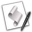## Math Routines

Here are some useful sub-routines addressing mathematical issues.

### Is a Passed Number an Odd Number?

This simple sub-routine will determine whether a passed whole number is even or odd. A returned value of false indicates the passed number is even, a returned value of true indicates the passed number is odd.A sub-routine for determining whether a passed numeric value is even or odd. The result is a boolean value of true (number is odd) or false (number is even):

on is_odd(this_number)
if this_number mod 2 is not 0 then
return true
else
return false
end if
end is_odd

Here's an example script using this sub-routine:A script demonstrating how to use the even/odd sub-routine:

repeat
display dialog "Enter an even integer:" default answer ""
try
if the text returned of the result is not "" then ¬
set the requested_number to the text returned of the result as integer
if is_odd(the requested_number) is false then exit repeat
end try
end repeat

on is_odd(this_number)
if this_number mod 2 is not 0 then
return true
else
return false
end if
end is_odd